##CS 660: Combinatorial Algorithms NP-Completeness

[To Lecture Notes Index]
San Diego State University -- This page last updated November 28, 1995## Reference

Computers and Intractability: A Guide to the Theory of NP-Completeness by Garey and Johnson, Chapters 1, 2, 3

## NP-Completeness

Intractable Problems
Problems which can not be solved in polynomial time

Causes of Intractability
Solution is too big
Generate all permutations of N
Problem is so hard it takes exponential time

Undecidable Problems
Problems which can not be solved
Halting Problem
Input: a program and input for program
Output: Will program halt on the input
Intractable and decidable Problems
1965 - First contrived example
1970's early - first natural example

P

All problems that can be solved in polynomial time

Decision Problems

Traveling Salesman Problem
Instance:
A finite set C = { c1, c2, ...., cm } of "cities"
A integer valued distance function d(ci, ck) >= 0
A bound B
Question:
Is there a tour of all the cities in C having total length no more than B?

Nondeterministic Algorithm

Two stages
Guessing Stage (the nondeterministic part)
Guess solution to a decision problem
Checking Stage (the deterministic part)
Checks to determine if the guess is correct

A nondeterministic algorithm for a decision problem is said to "solve" the problem in polynomial time if there is some guess, S, that leads the checking stage to verify that S is correct in polynomial time

NP

Class of decision problems that can be solved in polynomial time by a nondeterministic algorithm

Theorem. P is a subset of NP

Theorem. Let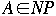, then there exists a polynomial p such that A can be solved by a deterministic algorithm having time complexity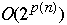Polynomial Transformations

f is a polynomial transformation from decision problem A to decision problem B if:
f maps potential solutions of A to potential solutions of B
f(x) can be computed in polynomial time
x is a solution to A if and only if f(x) is a solution to B

If there exists a polynomial transformation from A to B we write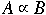Lemma. Assume that. If B is in P the A is in P.

Lemma. Ifand,then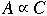Example

Let G = (V, E)

Simple Circuit
A sequence { v1, v2, ... , vk } of distinct vertices from V such that vn, vn+1 is an edge and
vk, v1 is an edge
Hamiltonian Circuit
Simple circuit that contains all vertices of G
Hamiltonian Circuit Problem (HC)

Instance: A graph G = (V, E)

Question: Does G contain a Hamiltonian circuit?
Traveling Salesman Problem (TS)

Instance:
A finite set C = { c1, c2, ...., cm } of "cities"
A integer valued distance function d(ci, ck) >= 0
A bound B

Question:
Is there a tour of all the cities in C having total length no more than B?

Theorem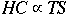NP-Complete

Polynomial Equivalent
A and B are polynomial equivalent if we haveand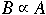A decision problem, A, is NP-Complete ifFor all decision problems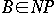we haveCook's Theorem

Let U = { u1, u2, ..., um } be a set of Boolean variables

Literals over U. Let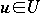"u" and "not u" are literals over U

Clause over U is a set of literals

A clause is satisfied if an assignment of True, False to U makes one member of the clause true

A collection, C, of clauses over U is satisfiable if and only if there exists some truth assignment for U that simultaneously satisfies all the clauses in C

Satisfiability
Instance:
A set U of variable and a collection C of clauses over U

Question:
Is C satisfiable

Cook's Theorem. Satisfiability is in NP-complete